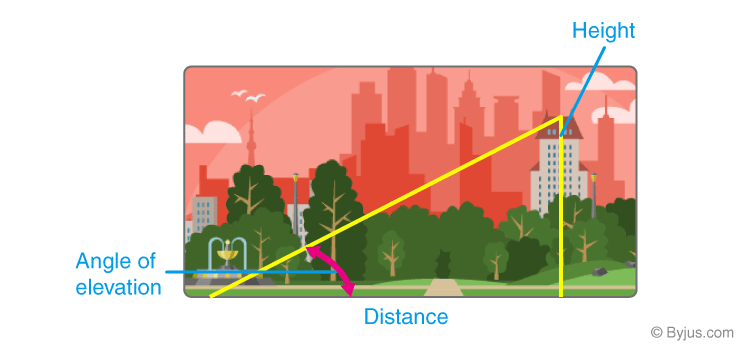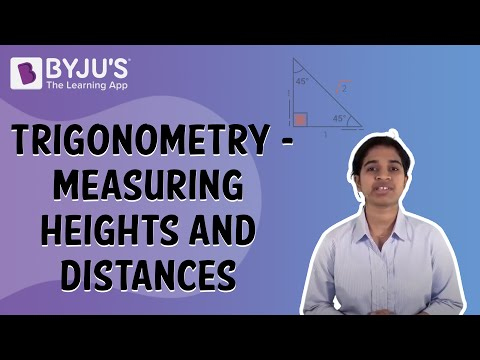# Applications of Trigonometry

Trigonometry can be defined as calculations with triangles involved in the study of lengths, heights and angles. Trigonometry and its functions have an enormous number of uses in our daily life. For instance, it is used in geography to measure the distance between landmarks, in astronomy to measure the distance of nearby stars and also in the satellite navigation system.

Before going into a detailed explanation of trigonometry applications, let’s start with the introduction of trigonometry and its functions.

## What is Trigonometry?

Trigonometry is a study of the relationship between angles, lengths and heights. It emerged in the third century BC involving applications from astronomy to geometric studies. Now it has spread its applications into wider fields like engineering, physics, surveying, architecture, astronomy and even in the investigation of a crime scene.

Apart from astronomy and geography, trigonometry is applicable in various fields like satellite navigation, developing computer music, chemistry number theory, medical imaging, electronics, electrical engineering, civil engineering, architecture, mechanical engineering, oceanography, seismology, phonetics, image compression and game development.

## Trigonometry Applications in Real Life

It may not have direct applications in solving practical issues but is used in various field. For example, trigonometry is used in developing computer music: as you are familiar that sound travels in the form of waves and this wave pattern, through a sine or cosine function for developing computer music. Here are a few applications where trigonometry and its functions are applicable.

### Trigonometry to Measure Height of a Building or a Mountain

Trigonometry is used in measuring the height of a building or a mountain. The distance of a building from the viewpoint and the elevation angle can easily determine the height of a building using the trigonometric functions.#### For More Information On Trigonometry – Measuring Heights And Distances, Watch The Below Video:Example:

If the distance from where the building is observed is 90 ft from its base and the angle of elevation to the top of the building is 35°, then find the height of the building.

Solution:

Given:

1. Distance from the building is 90 feet from its base.
2. The angle of elevation from to the top of the building is 35°.

Let us find the height of the building by recalling the trigonometric formulas. Here, the angle and the adjacent side length are provided. So, using the formula of tan.

$$\begin{array}{l} \tan 35^{\circ } = \frac{Opposite \; Side}{Adjacent \; Side} \end{array}$$

tan 35°h/90

90 × tan 35°

90 × 0.7002

= 63.018 ft

Thus, the height of the building is 63.018 ft.

### Trigonometry in Aviation

Aviation technology has evolved with many upgrades in the last few years. It has taken into account the speed, direction and distance as well as the speed and direction of the wind. The wind plays a vital role in when and how a flight will travel. This equation can be solved by using trigonometry.

For example, if an aeroplane is travelling at 250 miles per hour, 55° of the north of east and the wind blowing due to south at 19 miles per hour. This calculation will be solved using trigonometry and finding the third side of the triangle that will lead the aircraft in the right direction.

### Trigonometry in Criminology

Trigonometry is even used in the investigation of a crime scene. The functions of trigonometry are helpful to calculate a trajectory of a projectile and estimate the causes of a collision in a car accident. Further, it is used to identify how an object falls or at what angle the gun is shot.

### Trigonometry in Marine Biology

Trigonometry is often used by marine biologists for measurements to figure out the depth of sunlight that affects algae to photosynthesis. Using the trigonometric function and mathematical models, marine biologists estimate the size of larger animals like whales and also understand their behaviours.

Trigonometry is used in navigating directions; it estimates in what direction to place the compass to get a straight direction. With the help of a compass and trigonometric functions in navigation, it will be easy to pinpoint a location and also to find distance as well to see the horizon.

## Other Uses of Trigonometry

• The calculus is based on trigonometry and algebra
• The fundamental trigonometric functions like sine and cosine are used to describe the sound and light waves
• Trigonometry is used in oceanography to calculate the heights of waves and tides in oceans
• It used in the creation of maps
• It is used in satellite systems

Thus, here we have discussed Trigonometry and its importance along with the applications of this branch of mathematics in daily life, about which every student of Maths is expected to know.